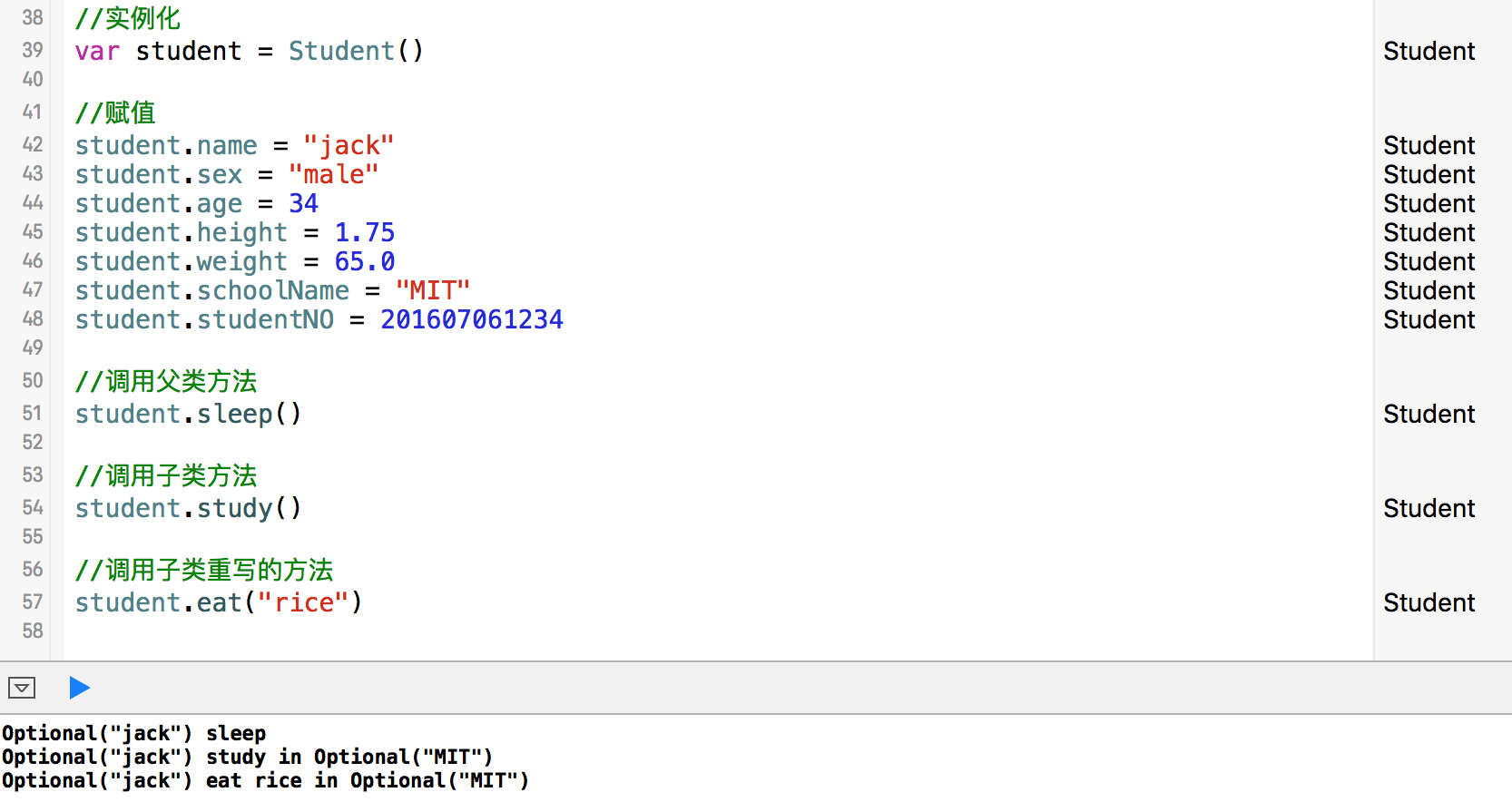# 父类的定义

``````class Person {

//定义类的属性
var name:String?
var sex:String = "Female"
var age:Int?
var height:Double?
var weight:Double?
var nationality:String = "China"

//定义类的方法
func sleep() {
print("\(name) sleep")
}

func eat(food:String) {
print("\(name) eat \(food)")
}

}
``````

# 子类的定义

``````class Student:Person {

var schoolName:String?
var studentNO:Int?

func study () {
print("\(name) study in \(schoolName)")
}

//重写父类的方法
override func eat(food: String) {
print("\(name) eat \(food) in \(schoolName)")
}
}
``````

# 子类的实例化与操作

``````//实例化
var student = Student()

//赋值
student.name = "jack"
student.sex = "male"
student.age = 34
student.height = 1.75
student.weight = 65.0
student.schoolName = "MIT"
student.studentNO = 201607061234

//调用父类方法
student.sleep()

//调用子类方法
student.study()

//调用子类重写的方法
student.eat("rice")
``````# 代码下载

https://github.com/99ios/SwiftBasic